# An Analytical Performance Evaluation of MapReduce Model Using Transient Queuing Model

An Analytical Performance Evaluation of MapReduce Model Using Transient Queuing Model

Chandra Sekhar DarapaneniBobba Basaveswara Rao Boggavarapu Bhanu Venkata Satya Vara Prasad Suneetha Bulla

Department of CSE, Acharya Nagarjuna University, Guntur 522510, India

University Computer Centre, Acharya Nagarjuna University, Guntur 522510, India

Department of Computer Science and Engineering, Koneru Lakshmaiah Education Foundation, Vaddeswaram, Guntur 522502, Andhra Pradesh, India

Corresponding Author Email:
dsekhar1807@gmail.com
Page:
46-53
|
DOI:
https://doi.org/10.18280/ama_b.641-407
25 June 2021
|
Accepted:
12 September 2021
|
Published:
31 Dcember 2021
| Citation

OPEN ACCESS

Abstract:

Today the MapReduce frameworks become the standard distributed computing mechanisms to store, process, analyze, query and transform the Bigdata. While processing the Bigdata, evaluating the performance of the MapReduce framework is essential, to understand the process dependencies and to tune the hyper-parameters. Unfortunately, the scope of the MapReduce framework in-built functions is limited to evaluate the performance till some extent. A reliable analytical performance model is required in this area to evaluate the performance of the MapReduce frameworks. The main objective of this paper is to investigate the performance effect of the MapReduce computing models under various configurations. To accomplish this job, we proposed an analytical transient queuing model, which evaluates the MapReduce model performance for different job arrival rates at mappers and various job completion times of mappers as well as the reducers too. In our transient queuing model, we appointed an efficient multi-server queuing model M/M/C for optimal waiting queue management. To conduct the experiments on proposed analytics model, we selected the Bigdata applications with three mappers and two reducers, under various configurations. As part of the experiments, the transient differential equations, average queue lengths, mappers blocking probability, shuffle waiting probabilities and transient states are evaluated. MATLAB based numerical simulations presented the analytical results for various combinations of the input parameters like λ, µ1 and µ2 and their effect on queue length.

Keywords:

transient queuing mode1, performance analytics, MapReduce, parallel computing, bigdata

1. Introduction

Since two decades, the advancements in mobile and internet technologies caused to generate huge volumes of data, termed as Bigdata. Hadoop is developed and implemented by the organizations with MapReduce programming model for processing the huge amount of data in parallel in the areas like data mining application, image processing and online applications like e-commerce etc. In reality, the organizations like Newyork stock exchange, Facebook and Google generates the petabytes of data periodically and processes that high volumes of data with adoption of the Hadoop MapReduce model. In this way the Hadoop MapReduce framework became a backbone for Bigdata processing.

Although MapReduce is efficient in Bigdata processing, while dealing the high dimensional data, MapReduce model has some limitations due to the network dependency and high bandwidth utilization. Some contemporary applications are running on the whole dataset instead of any sampled data set for preprocessing. This type of applications can be executed in parallel, on a number of machines in a Hadoop MapReduce paradigm. Today the popular search engines like Google, Amazon, and Baidu are daily running enormous number of MapReduce jobs on their data, with number of Hadoop clusters on their commodity computer clusters. As this MapReduce process is contains the high volumes of data and hardware clusters, it is a necessary to develop and analyze the performance models, in order to optimize and tuning the job parameters like mappers, reducers and buffer size, etc. Although the MapReduce frameworks have the inbuilt functionalities for performance evaluation, their scope is very limited in accessing the metrics required for us. Analytics are playing a significant role in metrics accessing, performance evaluation and even guides the process executor about the hyper parameters tuning to increase the performance. In this context several vendors and research have to pay attention to do research on designing and evolving the performance models for Hadoop Map-Reduce paradigm.

Since a decade, several researchers concentrated on designing and analyzing the performance of Map-Reduce models on Hadoop in clod environment through analytical, simulation and experimental models. The analytical models provide accurate and reliable estimates for performance metrics, when compared to the other models at a significantly lower cost. Yang et al.  developed an analytical model with job execution time and the throughput of the system as performance metrics and are validated under an experimental evaluation. Vianna et al. , designed an analytical performance model that combines the precedence graph and queuing network models for Hadoop workloads. Their research mainly focused on intra-job pipeline parallelism and validate with a simulation. Another Map-Reducing model on Hadoop is designed by Ke and Park , with analytical queuing model for optimizing the availability computing with derivation of the balancing equations. Apart from these, several other scholars also worked on analytics model design, but all of these works do not consider the time as one of the parameters in performance evaluation. These works also confirmed to deterministic service times.

In order to address the limitations in designing the efficient analytical model, in this paper we proposed an analytical transient queuing model, which evaluates the MapReduce model performance for different job arrival rates at mappers and various job completion times of mappers as well as the reducers too. In our transient queuing model, we appointed an efficient multi-server queuing model M/M/C  for optimal waiting queue management. This model will be help for implementing the optimal strategies to minimize the job execution time, efficient jobs scheduling, which leads to minimize the execution cost of the MapReduce.

The proposed analytical queuing model considers the time as a one of the parameters and derives the transient equations for computing the performance metrics. The main contributions of this paper are as follows:

1. Proposed an analytical transient queuing model for Hadoop MapReduce computations with the configuration of 3 mappers and 2 reducers.
2. Drawn the relevant transient state diagram for all possible cases.
3. Derived differential equations for finding the performance metrics like queue length and blocking probabilities of mappers and resumable probability of reducers in shuffle phase.
4. Numerical illustration is carried out with some examples and outlined the conclusions.

The remaining part of this paper is organized as follows. The relevant literature is presented precisely in Section 2 and the basics of the Map-Reduce model and the mathematical model, which is covering the generalized equations with all possible cases and the transitional state diagram, are explained in Section 3 and the numerical evaluation results and discussions of the proposed model are presented in Section 4. Conclusions and future scope of this work are given in Section 5.

2. Literature Survey

A lot of research has been carried out, in providing the solutions to the challenges  in handling the large volumes of data using the Map-Reduce framework. The parallel processing approach  is the core part of Big Data processing is implemented with the Map-Reduce technique. Former researchers [7-10] are focused on the research challenges and opportunities equipped with the Map-Reduce framework . Map-Reduce framework supports all types of data integration including structured, semi-structured and unstructured also. The researchers analyzed the behavior of Map-Reduce model with On Demand Integration (ODI) capabilities while processing the Bigdata .

Prasad et al.  concentrated on the performance and its related issues of Hadoop storage, processing and leverage capacity. According to Thanekar et al. , the performance improvement of Map-Reduce model can be possible with the enhancement of NameNode capabilities, which are used to reduce the work with bottlenecks management. Utilization of the dynamic schedulers  and job scheduling  helps in improving the performance of the MapReduce process. The performance can also be improved using the search space reduction process based on the patterns and user perspective . The mining and analytical techniques also helpful in recognition of patterns for improving search space and processing jobs cost reduction.

The performance metrics plays an important role in providing the assurance to the techniques for improving the performance of the Map-Reduce framework. Most of the former researchers [9, 10] focused on analyzing the system performance metrics by adopting different queuing models. Mak and Lundstrom , described an accurate and computationally efficient method for predicting the performance of a class of parallel computations, which are running on the concurrent systems. Yang et al. , described the performance model for better understanding of the impact of each component on overall program performance, and verified it using a small cluster. The results from these experiments indicated that their model can predict the performance of Map Reduce system and its relation with the configurations. Herodotou  presented the data ﬂow and the cost information at the ﬁne granularity of mapping and reducing phases of a job execution. These models can be used to estimate the performance of the MapReduce jobs and to ﬁnd the optimal conﬁguration settings to while running the jobs. Emanuel Vianna, Giovanni Comarela, Tatiana Pontes addressed the challenge of modeling Hadoop workloads, which can exhibit the intra-job precedence constraints and the synchronization delays. This approach extends the solutions based on a hierarchical model, where the execution flow of a parallel application is represented by a precedence tree and the contentions at the physical resources are captured by a closed queuing network . Salah and Calero  are aimed to investigate the analytical model by adopting queuing theory in data centers of the big data. The new queuing model developed the Map Reduce programming model accurately and discovered the nature of the programming model. The utilizations and mean waiting times of Mapper and Reducer are obtained from this model. The effect of the workload (number of Mapper slots) on the system performance (i.e., utilization) is presented with relevant statistics. Ke and Park , proposed model presented the failures and repairs of the map-reduce servers in detail.

From the analysis on the relevant literature, it is noted that the efforts of the researchers regarding to the analytical evaluation of MapReduce with queuing models, doesn’t considered the time factor in experimental evaluations. According to our knowledge, this transient queuing model approach does not adopted by any researcher in MapReduce analytics till date. Inspired from the former researches [4, 21] on transient queuing models, in this research to fulfill the research gaps, a transient finite server queuing model with infinite buffer assumption is adopted for investigating the performance of Hadoop MapReduce model. The following section describes the Hadoop MapReduce Transient Queuing model.

3. Hadoop Mapreduce Transient Queueing (HMRTQ) Model

In this paper, an attempt has been made to frame a model for MapReduce computing system by considering the M/M/C Transient queuing model where C is sum of number of mappers and number of reducers available in the system.

The proposed time dependent performance model is analyzed by the first order difference differential equations approach. To evaluate the dynamic behavior of the MapReduce model, a transient queuing analysis has been carried out for to find various performance metrics like average queue length, blocking probabilities of mappers and reducers. The basic architecture of the Hadoop MapReduce model is shown in the following Figure 1.

## 1.png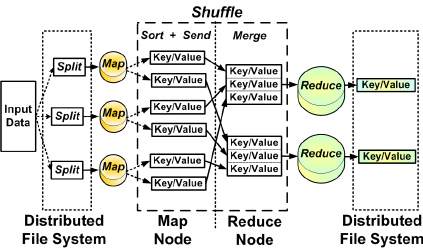Figure 1. Architecture of Hadoop MapReduce Model (REF)

The Hadoop MapReduce distributed processing is modeled as multi server queuing which is shown in Figure 2.

## 2.png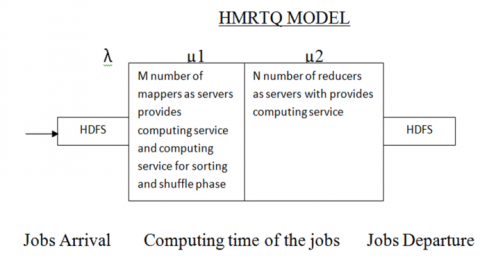Figure 2. Hadoop mapreduce distributed process model with multi server queuing

3.1 Assumptions

The total number of mappers and reducers are treated as the number of servers in process. Our queuing model is the finite server queuing model with specific number of mappers and reducers. For smooth running of our proposed system we made some assumptions are:

• The arrival rate of the job arrivals to the system follows the Poisson distribution.
• The completion times of both mappers and reducers follows the exponential distribution.
• The accepted jobs to the system are queued in FIFO manner.
• When all mappers are busy, the new jobs are not be considered for processing.
• The failures of mappers and reducers are not considered.
• HMRTQ has infinite capacity.

3.2 Model input parameters and performance metrics

When a new job arrives at HDFS, the splitting process at mappers will starts and the arrived job are moves on to the outgoing HDFS after completing the process of mapping, shuffling and reducing. In this work an assumption is made that the jobs are assumed to arrive at the HMRTQ and the job will get computing service in two phases are mappers along with shuffling and reduce phases. In general, the job arrival time and its processing time in MapReduce are vary over time, because of the dynamic change that takes place in internet traffic, bandwidth consumption and users’ behavior etc. So, analyzing the HMRTQ behavior as a function of time is the major challenge faced by the former researchers [5, 11]. This paper aimed to study and analyze the performance issues from the perspective of a transient queuing model design.

In our model, the intricacies associated with the complexity of the analytical model and the model considered here is confined to three mappers and two reducers. The relevant state transition diagram is presented in Figure 3 for M/M/5 transient queuing model of five servers (i.e. three mappers and two reducers). This diagram depicts the all possibilities of cases, that the state change from one to another, at the instance of a job arrival, engagement of servers (mappers and reducers) in handling of jobs and their respective completion. State diagram represents a state as (m.n) where m indicates the number of mappers engaged in executing the task given, and n indicates the number of reducers performing designated task after completion of tasks by mappers.

## 3.png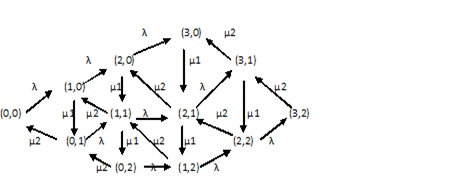Figure 3. State transition diagram for 3 Mappers and 2 reducers model

On the basis of the above state transition diagram, the following transitional differential equations are given for all possible cases at the time interval ‘t’.

Let Pm,n be the probability of ‘m’ mappers, which are busy in providing the service to the tasks at the rate of µ1 and ‘n’ represents the number of reducers involved in providing the service at the rate of µ2.

Case 1: When all mappers and all reducers are idle,

P10,0(t) = -(λ)P0,0(t)+μ2P0,1(t)         (1)

Case 2: When only one mapper is busy and the reaming mappers and reducers are free,

P11,0(t) = -(λ+ μ1) P1,0(t)+μ2P1,1(t)+ λ P0,0(t)        (2)

Case 3: When one mapper and one reducer is busy,

P11,1(t) = -(λ+ μ1+ μ2) P1,1(t) + μ1P1,0(t) + μ2 P1,2(t) +λ P0,1(t)        (3)

Case 4: When one reducer is busy and the remaining are idle,

P10,1(t) = -(λ+ μ2) P0,1(t) + μ1P1,0(t) + μ2 P0,2(t)        (4)

Case 5: When two mappers are busy,

P12,0(t) = -(λ+ μ1) P2,0(t) + μ2 P2,1(t) + λ P1,0(t)        (5)

Case 6: When two mappers and one reducer are busy,

P12,1(t) = -(λ+ μ1+ μ2) P2,1(t) + μ1P3,0(t) + μ2 P2,2(t) + λ P1,1(t)        (6)

Case 7: When two mappers and two reducers are busy,

P12,2(t) = -(λ+μ1+ μ2) P2,2(t)+μ1P3,1(t)+λP1,2(t)        (7)

Case 8: When one mapper and two reducers are busy,

P11,2(t) = -(λ+ μ1+ μ2) P1,2(t) +μ1P2,1(t) +λP0,2(t)        (8)

Case 9: When all reducers are busy and all mappers are idle,

P10,2(t) = -(λ+ μ2) P0,2(t) + μ1P1,2(t)        (9)

Case 10: When all mappers are busy and all reducers are idle,

P13,0(t) = -(μ1) P3,0(t) + μ2 P3,1(t) + λ P2,0(t)        (10)

Case 11: When all mappers are busy and only one reducer is busy,

P13,1(t) = -(μ1+ μ2)P3,1(t)+μ2 P3,2(t)+λP2,1(t)          (11)

Case 12: When all mappers and all reducers are busy,

P13,2(t)=-(μ1+μ2) P3,2(t)+λ P2,2(t)         (12)

The Average Queue Length, Blocking Probability of the mappers and Waiting Probability of shuffle Phase are presented based on the above derived transitional differential equations. These metrics are calculated for T time intervals, after that the averages are computed. The Probability generator function is defined as follows:

3.2.1 Average queue length of the system (L)

Depending on the inter-arrival times between the jobs and the completion time, the number of jobs in the system is varying from time to time. At any given time interval, the number of jobs under processing can be a random number.

The Average Queue Length is defined for the 3x2 MapReducer at the specific time ‘t’ is calculated as:

$L(t)=\sum_{i=0}^{3} \sum_{j=0}^{2}(i+j) P_{i, j} x^{i} y^{j}$

i.e. L(t)=0*p(0,0)+1*p(0,1)+2*p(0,2)+1*p(1,0)+2*p(1,1)+ 3*p(1,2)+2*p(2,0)+3*p(2,1)+4*p(2,2)+3*p(3,0)+4*p(3,1)+ 5*p(3,2)

$L=\sum_{t=1}^{T} L(t)$

3.2.2 Blocking probability of mappers or blocking probability of the system (BPm)

When all the mappers are busy in processing the jobs, the new arrival of the jobs are rejected by the system, due to the mapper does not allow the new jobs under overloading. The probability of number of jobs are rejected or blocked are treated as the Blocking Probability of Mappers. The Blocking Probability of Mappers will be:

$\begin{gathered} B P_{m}(t)=p(3,0)+p(3,1)+p(3,2) \\ B P_{m}=\sum_{t=1}^{T} B P_{m}(t) \end{gathered}$

3.2.3 Waiting probability of shuffle phase (WPs)

When all the reducers are busy in processing jobs completed by the mappers, the new or recent jobs completed by the mappers should wait in shuffle phase till the reducer become free. In this case, the probability of number of jobs is waiting in shuffle phase treated as the “Waiting Probability” of Shuffle Phase. It is calculated with the following formula.

$\begin{gathered} W P_{s}(t)=p(0,2)+p(1,2)+p(2,2)+p(3,2) \\ W P_{s}=\sum_{t=1}^{T} B P_{s}(t) \end{gathered}$

4. Numerical Evaluation

Numerical evaluation has been carried out, to assess the effect on MapReduce performance, for various combinations of the input parameters λ, µ1and µ2, where m=3 and n=2. For various transient values of ‘t’ from 0.2 to 1, with an interval of 0.2 (number of intervals T=5), the performance metrics and the averages are calculated with the help of the MATLAB numeric computing platform. The computational process can be divided in to three scenarios based on values of λ, µ1, and µ2, where one is varied and the other two are fixed. They are called as λ increasing, µ1 increasing and µ2 increasing scenarios. For all these scenarios, the performance metrics and their averages are calculated for different values of ‘t’ in range from 0.2 to 0, where m=3 and n=2.

4.1 λ increasing scenario

Oftenly the arrival rate of the jobs to the system are varied because of the user behavior, density of network traffic and availability of bandwidth etc. In this scenario, the metrics that are calculated for increasing values of λ and a fixed values of µ1 = 0.9 and µ2=0.8 for t in range from 0.2 to 1 with the incrimination of 0.2. The metrics are calculated for different values of λ ranging from 0.4 to 0.7 are presented in Table 1.

4.2 µ1 increasing scenario

Frequently the job completion rate at the mappers phase may be varied, because of the time taken for job computational time, i.e. the time will be low for short jobs and will be high for long jobs. In this scenario the metrics are calculated for increasing values of µ1 and the fixed values of λ=0.5 and µ2=0.8 for t in range from 0.2 to 1 with increment of 0.2. The metrics are calculated for different values of µ1, which is in range from 0.6 to 0.9, are presented in Table 2.

Table 1. Effect of various performance metrics for λ Increasing Scenario

 t 0.2 0.4 0.6 0.8 1 λ Queue Length L(t) L 0.4 0.0797 0.1575 0.2323 0.3033 0.3701 0.19048 0.5 0.0996 0.1969 0.2904 0.3792 0.4628 0.23815 0.6 0.1195 0.2362 0.3484 0.4549 0.5551 0.28568 0.7 0.1394 0.2756 0.4064 0.5304 0.6469 0.33312 Blocking Probability of Mappers BPm(t) BPm 0.4 0.0001 0.0005 0.0013 0.0026 0.0043 0.00147 0.5 0.0001 0.0009 0.0025 0.0048 0.0079 0.0027 0.6 0.0002 0.0015 0.0041 0.008 0.0128 0.00443 0.7 0.0004 0.0023 0.0062 0.012 0.0191 0.00667 Waiting Probability of Shuffle Phase WPs(t) WPs 0.4 0 0.0002 0.0007 0.0019 0.0038 0.0011 0.5 0 0.0003 0.0011 0.0029 0.0056 0.00165 0.6 0 0.0004 0.0016 0.0039 0.0076 0.00225 0.7 0 0.0005 0.0021 0.0051 0.0099 0.002933

Table 2. Effect of various performance metrics for µ1 Increasing Scenario

 t 0.2 0.4 0.6 0.8 1 µ1 Queue Length of the system L(t) L 0.6 0.0997 0.1978 0.2932 0.3851 0.4731 0.24148 0.7 0.0997 0.1975 0.2922 0.3831 0.4695 0.24033 0.8 0.0996 0.1972 0.2913 0.3811 0.4661 0.23922 0.9 0.0996 0.1969 0.2904 0.3792 0.4628 0.23815 Blocking Probability of Mappers BPm(t) BPm 0.6 0.0001 0.001 0.0028 0.0057 0.0096 0.0032 0.7 0.0001 0.0009 0.0027 0.0054 0.009 0.00302 0.8 0.0001 0.0009 0.0026 0.0051 0.0084 0.00285 0.9 0.0001 0.0009 0.0025 0.0048 0.0079 0.0027 Waiting Probability of Shuffle Phase WPs(t) WPs 0.6 0 0.0001 0.0005 0.0014 0.0029 0.000817 0.7 0 0.0002 0.0007 0.0019 0.0037 0.001083 0.8 0 0.0002 0.0009 0.0023 0.0047 0.00135 0.9 0 0.0003 0.0011 0.0029 0.0056 0.00165

Table 3. Effect of various performance metrics for µ2 Increasing Scenario

 t Time 0.2 0.4 0.6 0.8 1 µ2 Queue Length of the System L(t) L 0.6 0.0997 0.1978 0.2932 0.3851 0.473 0.241466 0.7 0.0997 0.1975 0.2922 0.3831 0.4694 0.240316 0.8 0.0996 0.1972 0.2913 0.3811 0.4661 0.239216 0.9 0.0996 0.1969 0.2904 0.3792 0.4629 0.238166 Blocking Probability of Mappers BPm (t) BPm 0.6 0.0001 0.0009 0.0026 0.0051 0.0084 0.00285 0.7 0.0001 0.0009 0.0026 0.0051 0.0084 0.00285 0.8 0.0001 0.0009 0.0026 0.0051 0.0084 0.00285 0.9 0.0001 0.0009 0.0026 0.0051 0.0084 0.00285 Waiting Probability of Shuffle Phase WPs(t) WPs 0.6 0 0.0002 0.001 0.0025 0.0051 0.001466 0.7 0 0.0002 0.0009 0.0024 0.0049 0.0014 0.8 0 0.0002 0.0009 0.0023 0.0047 0.00135 0.9 0 0.0002 0.0009 0.0023 0.0044 0.0013

4.3 µ2 increasing scenario

Timely, the job completion rate at the reducers phase also be varied due to irregularities in job computational time i.e. the time is less for the shorter jobs and is high for longer jobs. In this scenario, the metrics are calculated for increasing values of µ2, which is in range from 0.6 to 0.9 and the fixed values for λ=0.5 and µ1=0.8 and for ‘t’ the range is in 0.2 to 1 is presented in Table 3.

4.4 Time increasing scenario

Commonly, the job completion rate at the reducers phase can be varied, because of the variations in time taken for job execution. The computational time will be low for shorter jobs and it will be high for longer jobs. In this scenario the metrics are calculated for increasing values of t ranging from 0.2 to 1 and a fixed values of λ=0.5 and µ1=0.8 is.

Based on the aforementioned simulation results the following graphs are plotted for visual presentation of the results.

From Figure 4, It is observed that the queue lengths are increasing with the increase in values of parameter λ at fixed service rates of mappers and reducers. With the increase in task arrival rates, the system becomes busy in providing services, due to which the queue length increases. The growth rate of queue length shows a marginal decrease as arrival rate increases at a constant rate.

## 4.png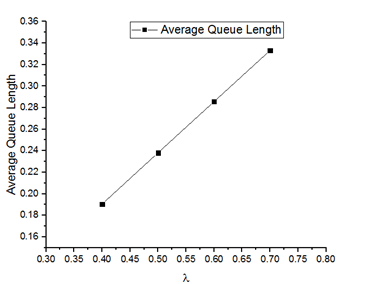Figure 4. State transition diagram for 3 Mappers and 2 Reducers Model

## 5.png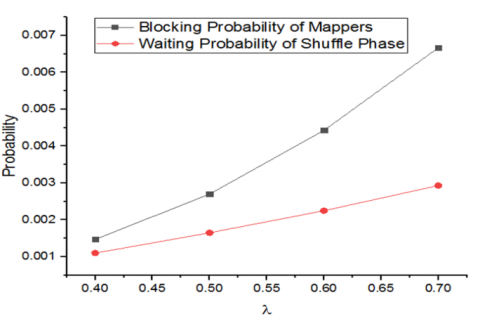Figure 5. Impact of Blocking probability of mappers and waiting probability of shuffle phase for λ Increasing Scenario

From Figure 5, we observed that the blocking probability of mappers is low initially and with the increase in job arrival rate, the blocking probability of mapper is high compared to reducer. This happend because the task should be completed by mapper first and subsequently to be done by reducers. It shows clearly that the blocking probabilities of mappers should be optimized more than reducer phase.

From Figure 6, the decrease in queue length is observed with the increase in service rate of mappers at fixed arrival rate of the tasks. It suits the practical issue of enhancing the service capacity of mappers, which completes tasks quickly and causes to decrease in queue length.

From Figure 7, It is observed that the decrease in blocking probability takes place when mappers service rate progresses and it also causes to the increase in blocking probability of reducers as the tasks finishes early with mappers.

## 6.png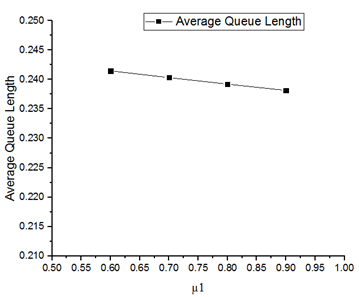Figure 6. Impact of average queue length for µ1 increasing scenario

## 7.pngFigure 7. Blocking probabilities of mappers and reducers at µ1Increasing Scenario

## 8.pngFigure 8. Impact of average queue length for µ2 increasing scenario

From Figure 8, we noticed a slight decrease in queue lengths with the increase in service rate (µ2), as the reducers server capacity used to finish the tasks, which are handed over from mappers.When a graph is plotted by considering mappers service rate on X-axis at fixed task arrival rate as 0.5 and fixed reducers service rate, µ2 as 0.8 can be shown as in Figure 9.

## 9.png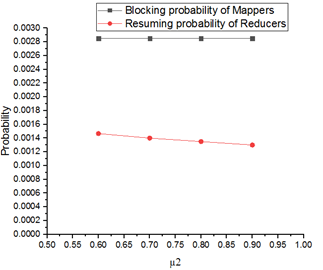Figure 9. Impact Blocking probability of mappers and Resuming probability of reducers at shuffle phase for µ2 Increasing Scenario

## 10.png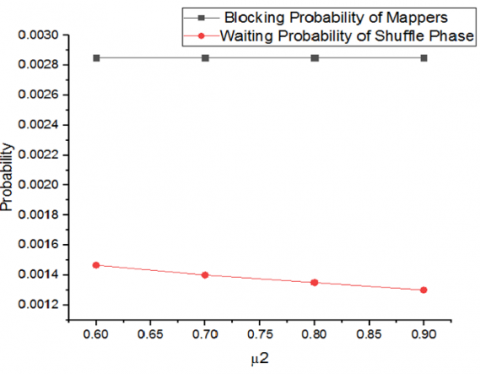Figure 10. Impact Blocking probability of mappers and Waiting probability at shuffle phase for µ2 Increasing Scenario

From Figure 10, we find that the time progresses blocking probability of mappers is constant as it doesn’t affected by the service rate changes of reducers. With the increase in service rates of reducers, the blocking probability of reducers decreases.

4.5 Influence of the time under progress

In this subsection, to investigate the behavior of the various metrics when time is in progress (from 0.2 to 1), where the other parameters λ, µ1 and µ2 has fixed values.

For this purpose the following graphs are plotted as per the metrics obtained in the Table I for various values of λ and the fixed values of µ1=µ2=0.8. Figure 11 is presenting that, the L will be increases when time is progress in straight line manner, which is true for all values of λ, when the mappers and reducers have the constant processing times. For the initial values of t i.e. when the beginning of the system the variation of L is too low, whereas the large values of t, i.e. the system is in running phase the significant of variation of L is identified. For high values of λ the L is also gets highest values.

Figure 12 is presenting that, the BPm will be increased when the time is in progress, which is true for all values of the λ, is happened when the mappers and reducers have the constant processing times. For the initial values of t i.e. when the beginning of the system the variation of BPm is low whereas the large values of ‘t’, i.e. the system is in running phase the significant of variation in BPm is identified. For high values of λ the BPm is also gets highest values.

## 11.pngFigure 11. Impact of the average queue length for different values of λ and fixed values of µ1 and µ2

## 12.png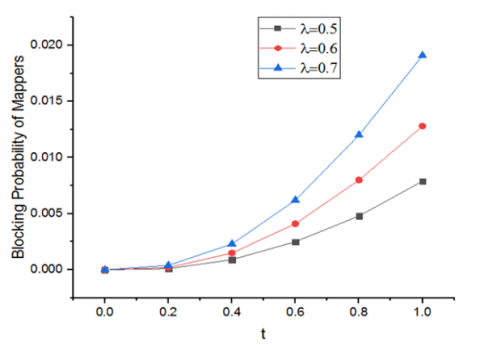Figure 12. Impact on average queue length for different values of the λ and the fixed values of µ1 and µ2

## 13.png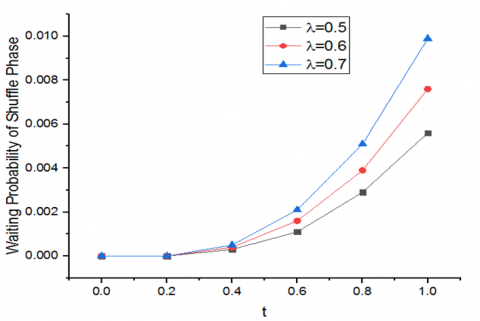Figure 13. Impact of the average queue length for different values of λ and fixed values of µ1 and µ2

From Figure 13, it is identified that, the WPs will be increases when time is in progress, which is true for all values λ, when the mappers and reducers have the constant processing times. For the initial values of ‘t’, i.e. when the beginning of the system the variation of WPs is low, whereas the large values of ‘t’ i.e. the system is in running phase the significant of variation in WPs is identified. For high values of λ the WPs is also gets highest values.

5. Conclusions

In this work, we proposed the transient analytical queuing model to address the limitations in performance modeling of the Hadoop MapReduce frameworks. This study investigated the impact of various performance metrics, for different values of job arrival rate and job completion times for both mappers and reducers based on time. The performance metrics Average Queue Length, Blocking Probability of Mappers and Waiting Probability of Shuffle Phase are calculated with time for the given input values of the job arrival rate, job completion times of the mappers and the reducers. The numerical experiments conducted using MATLAB on MapReduce, helped in calculating various metrics under different configurations of λ, µ1 and µ2 with time ‘t’. From the obtained results, it is noted that the queue length will do increases, when for fixed values of computational times.

In future, we are planning to expand the proposed analytical model to evaluate the dependencies among various metrics of map reduce framework. Apart from this we would like to customize resource utilization and job scheduling algorithms to achieve the high performance in queuing and processing the jobs.

References

 Yang, X., Sun, J. (2011). An analytical performance model of mapreduce. In 2011 IEEE International Conference on Cloud Computing and Intelligence Systems, Beijing, China, pp. 306-310. https://doi.org/10.1109/CCIS.2011.6045080

 Vianna, E., Comarela, G., Pontes, T., et al. (2013). Analytical performance models for MapReduce workloads. International Journal of Parallel Programming, 41(4): 495-525. https://doi.org/10.1007/s10766-012-0227-4

 Ke, Z., Park, N. (2017). Availability modeling and assurance of Map-Reduce computing. In 2017 IEEE 15th Intl Conf on Dependable, Autonomic and Secure Computing, 15th Intl Conf on Pervasive Intelligence and Computing, 3rd Intl Conf on Big Data Intelligence and Computing and Cyber Science and Technology Congress (DASC/PiCom/DataCom/CyberSciTech), pp. 965-970. https://doi.org/10.1109/DASC-PICom-DataCom-CyberSciTec.2017.160

 Braband, J. (1994). Waiting time distributions for M/M/N processor sharing queues. Stochastic Models, 10(3): 533-548. https://doi.org/10.1080/15326349408807309

 Thanekar, S.A., Subrahmanyam, K., Bagwan, A.B. (2016). Big data and mapreduce challenges, opportunities and trends. International Journal of Electrical & Computer Engineering, 6(6): 2911-2919. http://doi.org/10.11591/ijece.v6i6.pp2911-2919

 Brahmane, A.V., Murugan, R. (2018). Parallel processing on big data in the context of machine learning and hadoop ecosystem: A survey. International Journal of Engineering and Technology (UAE), 7(2.7): 577-588. http://dx.doi.org/10.14419/ijet.v7i2.7.10885

 Mak, V.W., Lundstrom, S.F. (1990). Predicting performance of parallel computations. IEEE Transactions on Parallel & Distributed Systems, 1(3): 257-270. https://doi.ieeecomputersociety.org/10.1109/71.80155

 Lin, X., Meng, Z., Xu, C., Wang, M. (2012). A practical performance model for hadoop mapreduce. In 2012 IEEE International Conference on Cluster Computing Workshops, Beijing, China, pp. 231-239. https://doi.org/10.1109/ClusterW.2012.24

 Xie, J., Yin, S., Ruan, X., et al. (2010). Improving mapreduce performance through data placement in heterogeneous hadoop clusters. In 2010 IEEE International Symposium on Parallel & Distributed Processing, Workshops and Phd Forum (IPDPSW), Atlanta, GA, pp. 1-9. https://doi.org/10.1109/IPDPSW.2010.5470880

 Polo, J., Carrera, D., Becerra, Y., Torres, J., Ayguadé, E., Steinder, M., Whalley, I. (2010). Performance-driven task co-scheduling for mapreduce environments. In 2010 IEEE Network Operations and Management Symposium-NOMS 2010, pp. 373-380. https://doi.org/10.1109/NOMS.2010.5488494

 Thanekar, S.A., Subrahmanyam, K., Bagwan, A.B. (2016). A study on MapReduce: Challenges and trends. Indonesian Journal of Electrical Engineering and Computer Science, 4(1): 176-183. http://dx.doi.org/10.11591/ijeecs.v4.i1.pp176-183

 Daya Sagar, K.V., Siva Nageswara Rao, G., Srikanth, T., Raghavendra, K. (2014). A relational analytic platform with Hadoop using the On Demand Integration (ODI) capability. International Journal of Applied Engineering Research, 9(13): 2095-2102.

 Prasad, G.S., Rajesh, P., Akram, S.W. (2018). A unified frame work to integrate hadoop and IOT to resolve the issues of storage, processing with leveraging capacity of analytics. International Journal of Engineering & Technology, 7(2.32): 147-149. http://doi.org/10.14419/ijet.v7i2.32.15390

 Thanekar, S.A., Bagwan, A., Subrahmanyam, K. (2017). Improving hadoop performance by enhancing name node capabilities. Frontiers, 6(2): 1-18. http://doi.org/10.21664/2238-8869.2017v6i2.p1-8

 Sai, V.S.V., Gowthami, K., Shyam, N.M.V.R., Komati, T.R., Gunasekhar, T., Sarada, N. (2017). A systematic survey on dynamic schedulers in hadoop. Journal of Advanced Research in Dynamical and Control Systems, 9(18).

 Nikhil, J., Vinod, T., Ramesh Kumar, M., Tenali, R.K. (2019). An efficient job scheduling in performance heterogeneous map reduce. International Journal of Recent Technology and Engineering, 8(1): 256-259.

 Satyanarayana, S., Jagadesh, B.N. (2016). Big data search space reduction based on pattern type and user perspective using mapreduce. International Journal of Pharmacy and Technology, 8(3): 17784-17792.

 Herodotou, H. (2011). Hadoop performance models. arXiv preprint arXiv:1106.0940.

 Lin, X., Meng, Z., Xu, C., Wang, M. (2012). A practical performance model for hadoop mapreduce. In 2012 IEEE International Conference on Cluster Computing Workshops, Beijing, China, pp. 231-239. https://doi.org/10.1109/ClusterW.2012.24

 Salah, K., Calero, J.M.A. (2013). Achieving elasticity for cloud MapReduce jobs. In 2013 IEEE 2nd International Conference on Cloud Networking (CloudNet), San Francisco, CA, USA, pp. 195-199. https://doi.org/10.1109/CloudNet.2013.6710577

 Sah, S.S., Ghimire, R.P. (2016). Transient analysis of queuing model. Journal of the Institute of Engineering, 11(1): 165. https://doi.org/10.3126/jie.v11i1.14711